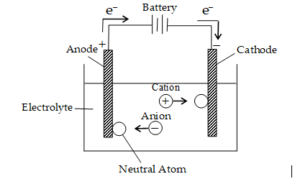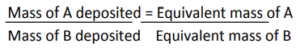# Electrolysis and Faraday’s Laws of ElectrolysisElectrolysis and Faraday’s Laws of Electrolysis

The process of decomposition of an electrolyte on passing an electric current through its aqueous solution or in the fused state is called electrolysis. An electrolyte (AB) when dissolved in water or when melted dissociates to produce corresponding ions (e.g., A+ and B). When the circuit is completed by closing the key, the following reactions occur at the two electrodes.

(a) The cations move towards the cathode. On reaching the cathode, they gain electrons (supplied by the battery) and thus become neutral atoms (At cathode) A+ + e —>  A (Reduction)(b)     The anions move towards the anode and on reaching the anode they lose electrons and convert to neutral atoms.
(At anode) B —> B + e (Oxidation)

Thus electrons from the source (battery) enter the solution (where they are taken up by cations) at the cathode and leave the solution at the anode. As a result, the flow of electricity continues along with the liberation of ions at the electrodes. Thus electrolysis involves oxidation (de-electronation of the anion) at the anode, and reduction (electronation) at the cathode.

Note that the above case is very simple where only dissociation of the salt is considered which is true when the electrolyte is taken in the molten state. However, when the aqueous solution of the electrolyte is taken, water also dissociates to a small extent as
H2O → H+ + OH

Thus in such cases, two different cations (H+ and A+) and two different anions (OH and B) are present in the solution. Of the two cations, that cation will be liberated at the cathode which has a higher reduction potential. Similarly, of the two anions, that anion will be discharged at the anode which has lower reduction potential. The process of electrolysis is further complicated when the electrodes are attacked by the ions of the electrolyte.

Faraday’s 1st law: The weight or amount of any substance produced during electrolysis is proportional to the amount of charge or electricity passed into the solution.
W α Q ⇒ W α it
W = zit
Where : z = electrochemical equivalent of substance deposited

Q = it
i = Q/t
i = dQ/dT

Faraday’s 2nd law: It states that when the same quantity of electricity is passed through solutions of different substances, the amount of substance deposited or liberated is directly proportional to their chemical equivalence. For e.g. when the same quantity of electricity is passed through solutions of two electrolytes A and B, then